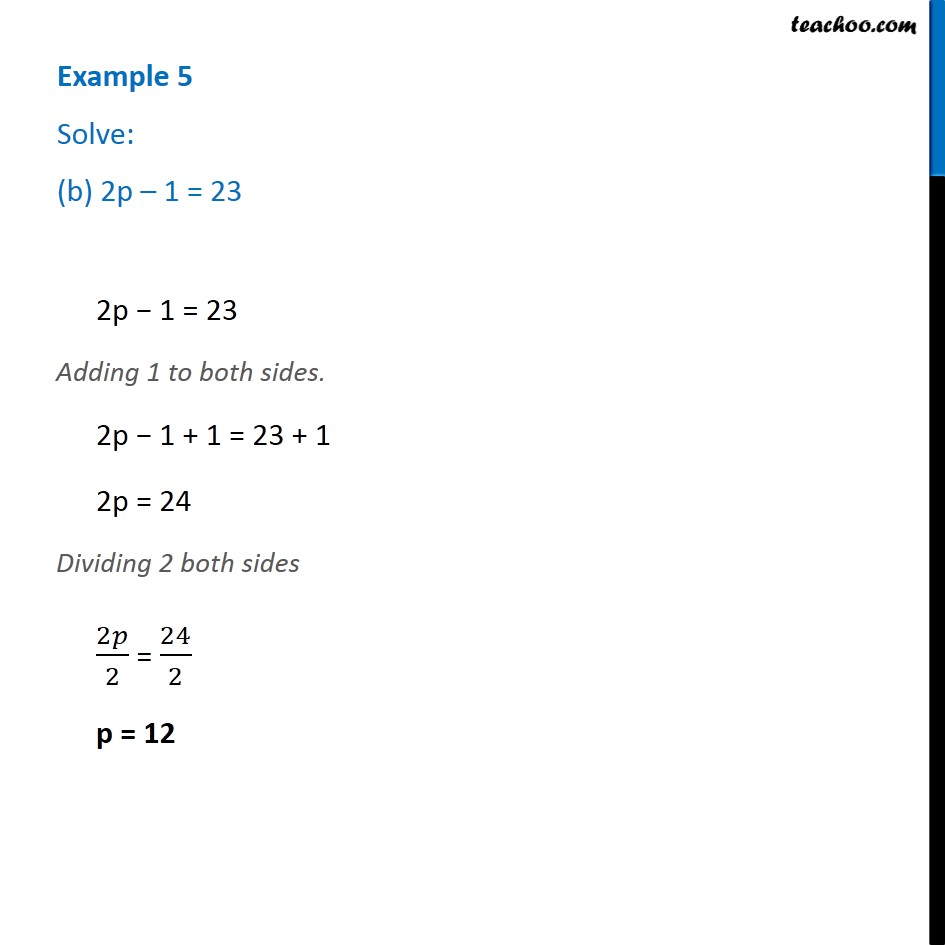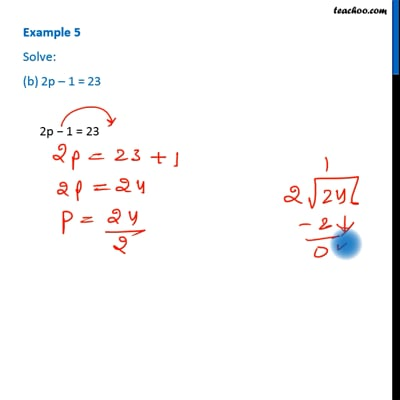Examples

Chapter 4 Class 7 Simple Equations
Serial order wiseThis video is only available for Teachoo black users

Get live Maths 1-on-1 Classs - Class 6 to 12

### Transcript

Example 5 Solve: (b) 2p – 1 = 23 2p − 1 = 23 Adding 1 to both sides. 2p − 1 + 1 = 23 + 1 2p = 24 Dividing 2 both sides 2𝑝/2 = 24/2 p = 12